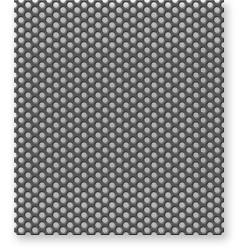# Resonant Sound Absorbers (Part VI)

### Example 11:

The next example (Example 5, p. 26) involved a resonator tuned to fR = 120 Hz; the available air depth was 4", the thickness of the sheet was V8", and it turned out that a hole diameter of V4", a percent open area of 0.4%, with only 0.081 holes per sq in satisfied the nomogram.

This time let us assume a value of R/pc = 2 for the filling; then the maximum absorption at resonance is (as we already know from the table on page 43):

Qrnax = 0.89.
The half-power bandwidth is:

6fH = 21r[1 + 2] (4!13560)(120)2
= 80 Hz.

Then

f1 = 120 -40 = 80 Hz
f2 = 120 + 40 = 160 Hz

and at these two frequencies, the absorption coefficient is 0.89/2 = 0.44. The entire frequency plot for this example is also given on Figure 27.

### "Self-Flow-Resistance" of Fine Perforated Metal Screens

We mentioned above, that, although it is desirable, from the point of view of trying to achieve high transparency from perforated sheet, to aim for tiny perforations closely spaced, there is a danger in over-doing it. First, if the sheet is painted, the fine holes may get clogged and this would spoil the transparency altogether.

Second, if the holes are fine enough, they will act like the fine pores in a glass fiber absorptive blanket, and may introduce unwanted sound absorption. This would be particularly undesirable if all we want from the perforated metal is acoustical transparency.

Therefore, it is a good idea, once you have finished your design for acoustical transparency, to follow through with a calculation of the self-resistance of the perforated sheet that you have chosen, and calculate the absorption coefficient of the sheet without any filling. This is explained below.

Considering that there is a large range of possible perforation patterns, there are two extremes that we could consider, in calculating the self-resistance of the sheet. It depends on whether the holes are wide or narrow, compared with the length of the so- called "viscosity waves" that cause the unwanted absorption.

Since the theory for coping with "in-between" situations is not developed, we must calculate both values and use the higher of the two results. In addition, there is an end correction to be taken into account, as in our calculations of resonance frequency, above.

So our self-resistance is given by:

Rself = Ro + 2~Ro, where the value for Ro is the larger of the two following formulas, depending on whether the flow resistance is dominated by (1) boundary layer effects or (2) laminar flow:

RoI = 4.24(b2tid3) v'f x 10-3 cgs rayls

or:

Ro2 = 2.88 (b2t/d4) X 10-3 cgs rayls

The value for 2~Ro is given by:

2~Ro = 4.19 (b2/d2) Vf x 10-3.

### Example 15:

As a more typical example of commonly used perforated sheet, let us repeat the calculation for Item #7 in Table 1 at a fre- quency of 8000 Hz. Here the hole diameter d is 0.063", the on-center spacing b of the holes is 0.125", and the sheet thickness t is 0.037". (Of the examples in Table 1, this one will yield the greatest self-resistance).

We find:

Ro1 = 0.88 cgs Rayls;
Ro2 = 0.11 cgs Rayls;
2~Ro = 1.48 cgs Rayls.

Again, Ro1 dominates.

Then:

R = 0.88 + 1.48 = 2.36;
R/pc = 0.58; and:
Xmax = 0.2.

As, suggested above, this value is about the highest that one would expect to find in typical perforated metal sheets. If the resonator were to be filled with any reasonable absorptive material, the acoustical performance would be governed by the filling, not by the self-resistance of the sheet.